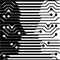MSc-IT Study MaterialJanuary 2011 Edition Computer Science Department, University of Cape Town| MIT Notes Home | Edition Home |

## Numbering

Numbering in a levelled set of diagrams is important, as the numbers help you to find your way around the levels. It is easily described by example. Suppose Receive Order is the process numbered 3 on the level one diagram (remember, numbers do not indicate any order, they are simply labels) and this is expanded to a level two diagram. The process numbers on the level two diagram will be 3.1, 3.2, 3.3 and so on. Suppose now that process 3.4 on the level two diagram is Register New Customer and needs further expansion to a level three diagram. The process numbers on this diagram will be 3.4.1, 3.4.2, 3.4.3 and so on. The rule used here is this: if X is the number of the process you wish to expand, then the numbers on the next level are X.1, X.2, X.3...

The same applies for data stores. Data stores that appear in a level two diagram expanding a process labelled 4 in the level one diagram will be numbered D4.1. D4.2, D4.3 and so on. Deeper levels will be D4.1.1, D4.1.2, the numbering scheme being just the same as for processes.

Note though, it is not the data stores that are expanded. They may simply appear in the expansion of a process.